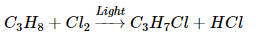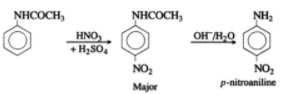Courses

# Test: Chemistry Mock- 8

## 45 Questions MCQ Test NEET Mock Test Series | Test: Chemistry Mock- 8

Description
This mock test of Test: Chemistry Mock- 8 for NEET helps you for every NEET entrance exam. This contains 45 Multiple Choice Questions for NEET Test: Chemistry Mock- 8 (mcq) to study with solutions a complete question bank. The solved questions answers in this Test: Chemistry Mock- 8 quiz give you a good mix of easy questions and tough questions. NEET students definitely take this Test: Chemistry Mock- 8 exercise for a better result in the exam. You can find other Test: Chemistry Mock- 8 extra questions, long questions & short questions for NEET on EduRev as well by searching above.
QUESTION: 1

Solution:
QUESTION: 2

Solution:
QUESTION: 3

### If the dipole moments of toluene and nitrobenzene are 0.43 D and 3.93 D then what is the expected dipole moment of p-nitrotoluene?

Solution:
QUESTION: 4

A element M has an atomic mass 19 and atomic number 9. Its ion is represented by

Solution:
QUESTION: 5
The addition of HCN to a carbonyl compound is an example of
Solution:
QUESTION: 6
Which of the following element is responsible for oxidation of water to O₂ in biological processes?
Solution:
QUESTION: 7

The hybridisation of Ag in the linear complex [Ag(NH₃)₂]⁺ is

Solution:
QUESTION: 8
For reaction at equilibrium, Le Chatelier's principle predicts the effects of
Solution:
QUESTION: 9

For melting of a solid at 25oC ,the fusion process requires energy equivalent to 2906 Joules to be added to system considering the process to be reversible at fusion point ,the entropy change of the process is

Solution:
QUESTION: 10
The order of a reaction of a radioactive decay is
Solution:
QUESTION: 11
Evaporation of water is
Solution:
QUESTION: 12
Which of the following is used as an antiseptic?
Solution:
QUESTION: 13
IUPAC name of CH₂=CH-CN is
Solution:
QUESTION: 14
Two elements whose electronegativities are 1.2 and 3.0, the bond formed between them would be
Solution: When the electronegativity difference is more than 1.7, the bond formed will be ionic in nature.
QUESTION: 15
The IUPAC name of K₃[Ir(C₂O₄)₃] is
Solution:
QUESTION: 16
Which lanthanide compound, after dissolving in selenium oxychloride, is used as a powerful liquid lasers?
Solution:
QUESTION: 17
Which of the following reactions actually take place?
Solution:
QUESTION: 18
In many respects lithium differs from other alkali metals. The main reason for this is
Solution:
QUESTION: 19

The rms velocity of an ideal gas at 27oC is 0.3 ms⁻1. Its rms velocity at 927oC (in ms⁻1) is

Solution:
QUESTION: 20
The alkali metal that reacts with nitrogen directly to form nitride is
Solution:
QUESTION: 21
Which of the following processes does not involve a catalyst?
Solution:
QUESTION: 22
Silica is soluble in
Solution:
QUESTION: 23
Which one of the following is triple super phosphate
Solution:
QUESTION: 24
Electron affinity of sulphur is
Solution:
QUESTION: 25
When cold NaOH reacts with Cl₂ which of the following is formed?
Solution:
QUESTION: 26
Plaster of Paris hardens by
Solution:
QUESTION: 27The above reaction is an example of

Solution:
QUESTION: 28
Which of the following sulphides has the lowest solubility product ?
Solution:
QUESTION: 29
Water can be tested by
Solution:
QUESTION: 30
The reason for geometrical isomerism by 2-butene is
Solution:
QUESTION: 31
Which responds carbylamine reaction?
Solution:
QUESTION: 32

In the following, electrophile is:
HO ― NO2

Solution:
QUESTION: 33

Acetanilide on nitration followed by alkaline hydrolysis mainly gives

Solution:QUESTION: 34
The molecule with maximum boiling point is
Solution:
QUESTION: 35
The type of isomerism exhibited by pentamminenitro chromium (III) chloride is
Solution:
QUESTION: 36
Which of the following has the highest melting point?
Solution: The diatomic molecules of halogens are held together by weak van der waal's force of attraction. The strength of these van der waal's forces increases as the size of the halogen increases from fluorine to chlorine. As a result, F2 and Cl2 are gases, Br2 is a liquid and I2 is solid. Hence, as we move down the group, the melting points and boiling points increases with atomic number from fluorine to iodine.
QUESTION: 37
Soft drinks and baby feeding bottles are generally made up of
Solution:
QUESTION: 38
A compound with empirical formula CH₂O has a vapour density of 30. Its molecular formula is
Solution:
QUESTION: 39

In acidic medium, one mole of MnO₄ accepts how many moles of electrons in a redox process ?

Solution:
QUESTION: 40
Which of the following metal oxides is antiferromagnetic in nature?
Solution:
QUESTION: 41
How much water is needed to dilute 10ml of 10 N hydrochloric acid to make it exactly decinormal (0.1N)?
Solution:
QUESTION: 42
Optical isomerism is shown by:
Solution:
QUESTION: 43

When 10 g of 90% pure limestone is heated completely, the volume (in litres) of carbon dioxide liberated at STP is

Solution:
QUESTION: 44
Term catalyst was given by
Solution:
QUESTION: 45
The highest oxidation state in transition metals is
Solution: